人工智能
• l一般情况下机器人各关节的几何关系可通过Denavit-Hartenberg(D—H)参数表来描述。 提出了采用D—H参截袁生成机器人三堆虚拟模型的方法，实现了机器人的可视 匕 仿真系统提供 的视图控制功能和场景漫游功能实现了多...
• 2019/5/30 新编辑：已经有两个小伙伴证明了：我这个程序在我的MATLAB2017b上运行没问题，但在2018版本上就会报错，我也不知道是什么原因！！！ 2019/4/25新编辑：改正了zhengyundongxue和niyundon...


2020/7/22补充：我把所有文件和机器人工具箱打包了，新来的朋友可以不往下看了，直接下载用吧。

链接：https://pan.baidu.com/s/

1n7uyCeUrw1zO1b7UGNDSnw

%%%%%%%%%%%%%%%%%%%%%自行把上面链接放到一行，进行搜索%%%%%%%%%%%%%%%%%%%%%
提取码：csdn

2020/7/22 补充：用机器人工具箱迭代求逆解有时报错，但niyundongxue.m求逆解没问题。所以有两种方法解决：1. 注释掉工具箱求逆解过程:

%  AA=ikine(robot_UR5,A);

2. 更换下theta角度值，比如更换为:

theta=[1,1/2,1/3,1,1,1];%没有什么含义，随便取的

此博客目的在于写个能求正逆解的MATLAB程序，便于其他功能开发，比较速成，被验证可行。

2019/5/30 新编辑：已经有两个小伙伴证明了：我这个程序在我的MATLAB2017b上运行没问题，但在2018版本上就会报错，我也不知道是什么原因！！！

2019/4/25 新编辑：改正了zhengyundongxue和niyundongxue中的DH表（之前写错了，汗。。。）

背景介绍：

本人想用    MATLAB+V-REP  仿真机器人，对机器人的正逆运动学简单写了下代码实现部分。

首先是正运动学求解代码（所用DH参数表为UR5）

function T = zhengyundongxue(theta)
%已知关节角求变换矩阵
a=[0,-0.42500,-0.39225,0,0,0];
d=[0.089159,0,0,0.10915,0.09465,0.08230];
alpha=[pi/2,0,0,pi/2,-pi/2,0];

T01=T_para(theta(1),d(1),a(1),alpha(1));
T12=T_para(theta(2),d(2),a(2),alpha(2));
T23=T_para(theta(3),d(3),a(3),alpha(3));
T34=T_para(theta(4),d(4),a(4),alpha(4));
T45=T_para(theta(5),d(5),a(5),alpha(5));
T56=T_para(theta(6),d(6),a(6),alpha(6));

T=T01*T12*T23*T34*T45*T56;

end

function T = T_para(theta,d,a,alpha)
T=[ccc(theta),-sss(theta)*ccc(alpha),sss(theta)*sss(alpha),a*ccc(theta);
sss(theta),ccc(theta)*ccc(alpha),-ccc(theta)*sss(alpha),a*sss(theta);
0,sss(alpha),ccc(alpha),d;
0,0,0,1];
end

好了，问题来了，这个ccc和sss函数就是cos和sin，我承认我SB了。。。其实我的目的是，在角度和弧度之间切换。

function sss=sss(a)
%     sss=sin(a/180*pi);
sss=sin(a);
end

function ccc=ccc(a)
%     ccc=cos(a/180*pi);
ccc=cos(a);
end

至此，正运动学代码部分贴完了。

其次，是逆运动学代码部分

function theta=niyundongxue(T)
%变换矩阵T已知
%SDH:标准DH参数表求逆解（解析解）
%部分DH参数表如下，需要求解theta信息

a=[0,-0.42500,-0.39225,0,0,0];
d=[0.089159,0,0,0.10915,0.09465,0.08230];

alpha=[pi/2,0,0,pi/2,-pi/2,0];% alpha没有用到,故此逆解程序只适合alpha=[pi/2,0,0,pi/2,-pi/2,0]的情况！

nx=T(1,1);ny=T(2,1);nz=T(3,1);
ox=T(1,2);oy=T(2,2);oz=T(3,2);
ax=T(1,3);ay=T(2,3);az=T(3,3);
px=T(1,4);py=T(2,4);pz=T(3,4);

%求解关节角1
m=d(6)*ay-py;  n=ax*d(6)-px;
theta1(1,1)=atan2(m,n)-atan2(d(4),sqrt(m^2+n^2-(d(4))^2));
theta1(1,2)=atan2(m,n)-atan2(d(4),-sqrt(m^2+n^2-(d(4))^2));

%求解关节角5
theta5(1,1:2)=acos(ax*sin(theta1)-ay*cos(theta1));
theta5(2,1:2)=-acos(ax*sin(theta1)-ay*cos(theta1));

%求解关节角6
mm=nx*sin(theta1)-ny*cos(theta1); nn=ox*sin(theta1)-oy*cos(theta1);
theta6=atan2(mm,nn)-atan2(sin(theta5),0);

%求解关节角3
mmm=d(5)*(sin(theta6).*(nx*cos(theta1)+ny*sin(theta1))+cos(theta6).*(ox*cos(theta1)+oy*sin(theta1))) ...
-d(6)*(ax*cos(theta1)+ay*sin(theta1))+px*cos(theta1)+py*sin(theta1);
nnn=pz-d(1)-az*d(6)+d(5)*(oz*cos(theta6)+nz*sin(theta6));
theta3(1:2,:)=acos((mmm.^2+nnn.^2-(a(2))^2-(a(3))^2)/(2*a(2)*a(3)));
theta3(3:4,:)=-acos((mmm.^2+nnn.^2-(a(2))^2-(a(3))^2)/(2*a(2)*a(3)));

%求解关节角2
mmm_s2(1:2,:)=mmm;
mmm_s2(3:4,:)=mmm;
nnn_s2(1:2,:)=nnn;
nnn_s2(3:4,:)=nnn;
s2=((a(3)*cos(theta3)+a(2)).*nnn_s2-a(3)*sin(theta3).*mmm_s2)./ ...
((a(2))^2+(a(3))^2+2*a(2)*a(3)*cos(theta3));
c2=(mmm_s2+a(3)*sin(theta3).*s2)./(a(3)*cos(theta3)+a(2));
theta2=atan2(s2,c2);

%整理关节角1 5 6 3 2
theta(1:4,1)=theta1(1,1);theta(5:8,1)=theta1(1,2);
theta(:,2)=[theta2(1,1),theta2(3,1),theta2(2,1),theta2(4,1),theta2(1,2),theta2(3,2),theta2(2,2),theta2(4,2)]';
theta(:,3)=[theta3(1,1),theta3(3,1),theta3(2,1),theta3(4,1),theta3(1,2),theta3(3,2),theta3(2,2),theta3(4,2)]';
theta(1:2,5)=theta5(1,1);theta(3:4,5)=theta5(2,1);
theta(5:6,5)=theta5(1,2);theta(7:8,5)=theta5(2,2);
theta(1:2,6)=theta6(1,1);theta(3:4,6)=theta6(2,1);
theta(5:6,6)=theta6(1,2);theta(7:8,6)=theta6(2,2);

%求解关节角4
theta(:,4)=atan2(-sin(theta(:,6)).*(nx*cos(theta(:,1))+ny*sin(theta(:,1)))-cos(theta(:,6)).* ...
(ox*cos(theta(:,1))+oy*sin(theta(:,1))),oz*cos(theta(:,6))+nz*sin(theta(:,6)))-theta(:,2)-theta(:,3);

end

代码测试部分：


L1 = Link('d', 0.089159, 'a', 0,        'alpha', pi/2 ,'standard' );
L2 = Link('d', 0,        'a', -0.42500,   'alpha',   0  ,'standard' );
L3 = Link('d', 0,        'a', -0.39225, 'alpha',   0  ,'standard' );
L4 = Link('d', 0.10915,  'a', 0,        'alpha', pi/2 ,'standard' );
L5 = Link('d', 0.09465,  'a', 0,        'alpha', -pi/2 ,'standard');
L6 = Link('d', 0.08230,  'a', 0,        'alpha',   0   ,'standard');

robot_UR5.teach(); %可以自由拖动的关节角度

theta=[1,1/2,1,1,1,1];%没有什么含义，随便取的

%利用机器人工具箱求正、逆运动学
A=fkine(robot_UR5,theta);
AA=ikine(robot_UR5,A);

%利用刚写的函数求正、逆运动学
B=zhengyundongxue(theta);
BB=niyundongxue(B);

结果部分：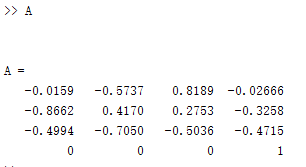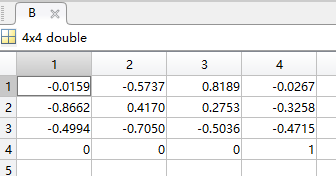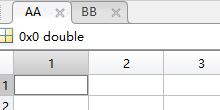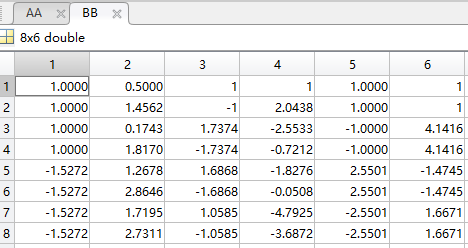经再次运行正运动学验证，我所求的8组逆解都是正确的。（不知道为什么机器人工具箱没有求出逆解？？？）

Tip：

2. 关于奇异位置，未作说明。

3. 代码有没有硬核？名字无所谓，瞎起的，代码的逻辑不知道别人能不能看懂，能用就完事了。

4. 改进DH参数表求逆运动学，请参考https://blog.csdn.net/jldemanman/article/details/80785075，本人未验证她的代码是否可用（明天周五了，想了想这周进度，又是深深的难过。。。）

展开全文• 在MATLAB中利用符号函数功能，通过手动输入DH参数符号矩阵 or Excel文件导入DH参数表从而获得DH符号矩阵公式1.前言2.废话不多说，上代码演示效果1. **函数使用方法一：==通过excel直接导入DH参数获得DH矩阵==**2. **...
在MATLAB中利用符号函数功能，通过手动输入DH参数符号矩阵 or Excel文件导入DH参数表从而获得DH符号矩阵公式1.前言2.废话不多说，上代码演示效果1. **函数使用方法一：==通过excel直接导入DH参数获得DH矩阵==**2. **函数使用方法二：==将DH参数数据通过手动建立一个符号矩阵输入==**注：本次利用到了matlab符号函数计算工具，具体的相关知识参考：
1.前言
由于自己的专业方向，现在正在开始学习机器人相关知识。在机器人领域中（特别是机械臂之类的工业机器人），如果需要对机器人进行运动学分析，我们常常需要用到D-H矩阵（即Denavit-Hartenberg矩阵）。
然而求过DH矩阵的小伙伴都知道，我们目前基本都是通过对应的DH参数表以及DH矩阵计算公式手动计算出各个DH转换矩阵。特别是在获得DH矩阵之后一般还需进行多个DH转换矩阵的相乘等操作，从而获得我们所需的变换关系。如果我们自己手动去计算这些矩阵，实在是恶心…
考虑到人工手动计算DH矩阵的麻烦，我便想“能不能利用工具，只要我输入DH参数就能自动算出对应的DH矩阵？”
今天，我在这里将要分享给大家的是一个自己写的  matlab  函数，这个函数便是用来求DH矩阵的。函数部分介绍如下：
%%%%%%%%%%%%%%%%%%%%%%%%%%%%%%%%%%%%%%%%%%%%%%%%%%%%%%%%%%%%%%%%%%%%%%%%%%%
%                     符号矩阵计算 DH 转换矩阵公式
% 1、函数功能：[Dof,A] = m_SigalMesu(DH_table_or_excelname,sheetname,range)
%    利用符号矩阵 or excel文件导入 D-H 参数表，自动获得转换矩阵
%    DH_table_or_excelname —— DH参数符号矩阵 or 具有DH参数便表的excel文件名
%    sheetname —— 只有在excel导入时候才有作用，它表示导入数据在excel文件的sheetname sheet中
%    range —— 只有在excel导入时候才有作用，它表示DH参数表范围
%    Dof —— 转换矩阵个数
%    A   —— 转换矩阵，三维数据。A(:,:,i)为求得的第i个DH矩阵
%
% 2、D-H参数输入格式：
%    [连杆1长度a1 连杆1扭角aerf1 关节1偏移量d1 关节1角度theta1;
%     连杆2长度a2 连杆2扭角aerf2 关节2偏移量d2 关节2角度theta2;
%                            ....                            ]
%    符号矩阵直接创建方法：
%    1）M = sym('[a,2*b;3*a,0]');
%    2) M = str2sym('[a,2*b;3*a,0]');
%  注：sym函数可能不再适用
%
% 3、从excel导入输入格式：
%    [Dof,A] = m_SigalMesu('excelname','sheetname','range')
%    如：[Dof,A] = m_SigalMesu('dh_test.xlsx','sheet1','B2:E8')
%
%  注：该函数如果输入一个参数，则表示输入为DH参数符号矩阵；
%      如果需要从excel导入，则需要根据自己建立的参数表输入：
%      excel文件名、sheet名、甚至对应DH参数范围
%%%%%%%%%%%%%%%%%%%%%%%%%%%%%%%%%%%%%%%%%%%%%%%%%%%%%%%%%%%%%%%%%%%%%%%%%%%
function [Dof,A] = m_SigalMesu(DH_table_or_excelname,sheetname,range);

函数部分截图如下：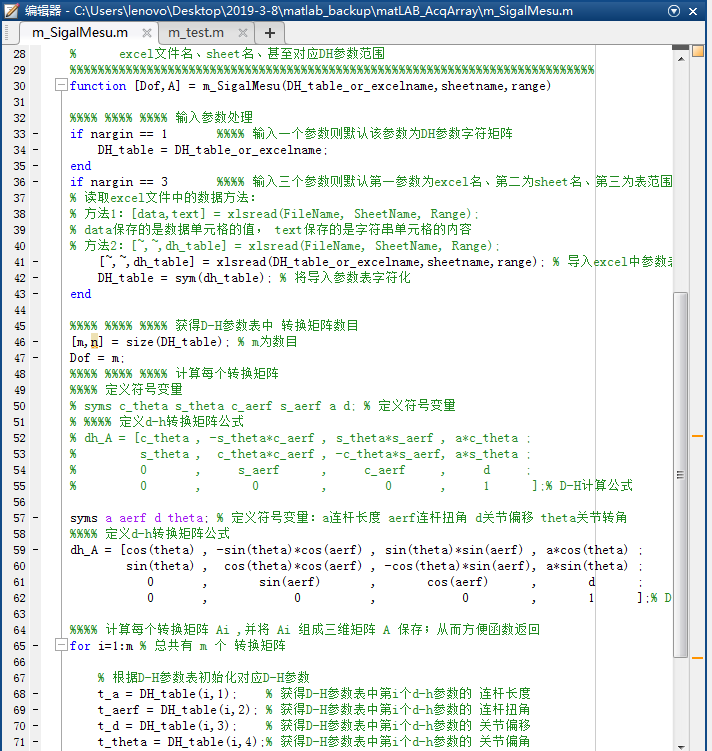并且这样求得的矩阵还有个好处：利用matlab的符号运算功能，将获得的DH矩阵，能够直接通过matlab用于计算我们所需的变换矩阵！！！——从而不再需要我们自己手动计算   ~~~
由于创作不易，具体函数代码，大家如果需要，则到我主页下载；或留言邮箱~~~
2.废话不多说，上代码演示效果
1. 函数使用方法一：通过excel直接导入DH参数获得DH矩阵
1）、建立一个excel文件，并将自己的DH参数按照固定格式（由于我在建这个函数时将格式给固定了，因此使用这函数时输入格式要按照固定格式来）输入到excel表中。如下例子：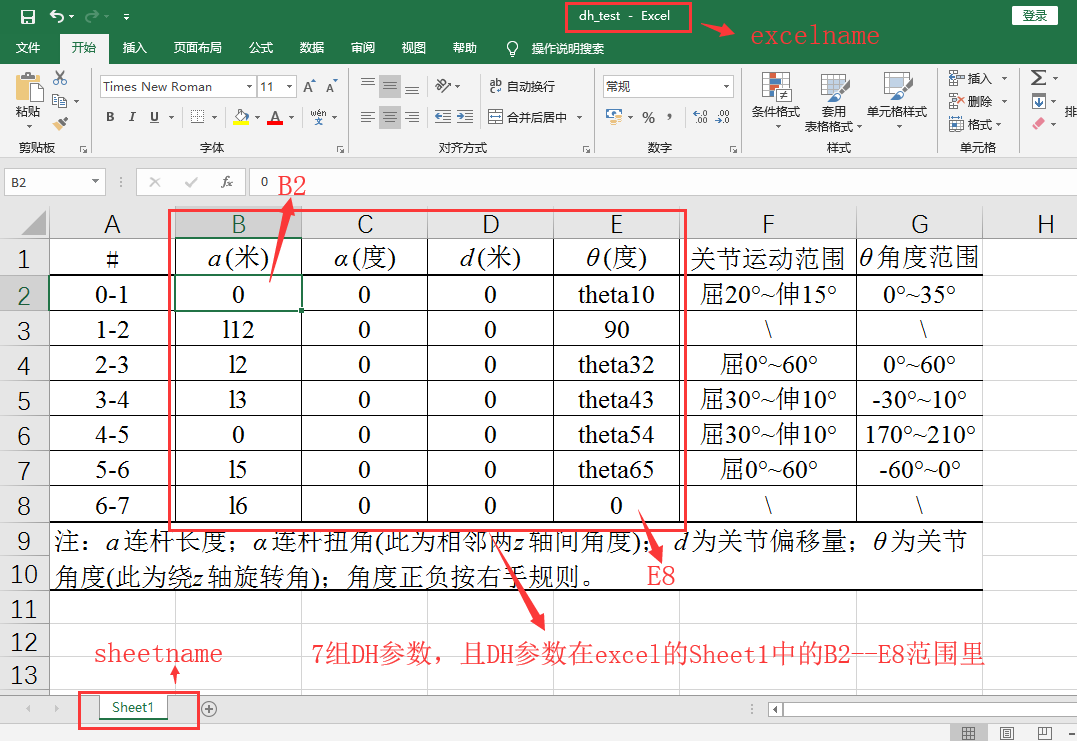2）、直接调用函数，输入excel名、sheet名、表格范围
%%%% 从excel文件中导入数据，并求得DH矩阵
>>[Dof,A] = m_SigalMesu('dh_test.xlsx','sheet1','B2:E8') % 输入参数为：excel文件名、文件中对应的sheet名、表范围

Dof =

7   % DH矩阵个数

A(:,:,1) =

[ cos(theta10), -sin(theta10), 0, 0]
[ sin(theta10),  cos(theta10), 0, 0]
[            0,             0, 1, 0]
[            0,             0, 0, 1]  % 第1个DH矩阵函数

A(:,:,2) =

[ cos(90), -sin(90), 0, l12*cos(90)]
[ sin(90),  cos(90), 0, l12*sin(90)]
[       0,        0, 1,           0]
[       0,        0, 0,           1] % 第2个DH矩阵函数

A(:,:,3) =

[ cos(theta32), -sin(theta32), 0, l2*cos(theta32)]
[ sin(theta32),  cos(theta32), 0, l2*sin(theta32)]
[            0,             0, 1,               0]
[            0,             0, 0,               1] % 第3个DH矩阵函数

A(:,:,4) =

[ cos(theta43), -sin(theta43), 0, l3*cos(theta43)]
[ sin(theta43),  cos(theta43), 0, l3*sin(theta43)]
[            0,             0, 1,               0]
[            0,             0, 0,               1]

A(:,:,5) =

[ cos(theta54), -sin(theta54), 0, 0]
[ sin(theta54),  cos(theta54), 0, 0]
[            0,             0, 1, 0]
[            0,             0, 0, 1]

A(:,:,6) =

[ cos(theta65), -sin(theta65), 0, l5*cos(theta65)]
[ sin(theta65),  cos(theta65), 0, l5*sin(theta65)]
[            0,             0, 1,               0]
[            0,             0, 0,               1]

A(:,:,7) =

[ 1, 0, 0, l6]
[ 0, 1, 0,  0]
[ 0, 0, 1,  0]
[ 0, 0, 0,  1]

>>

Dof——为DH参数组的数目，也是DH矩阵的个数；
A——便是返回的DH参数的集合；它是一个三维数据，因而A(:,:,i)就是第i个DH矩阵。
这样一个求DH矩阵的函数，只要输入了 DH参数 就能简简单单的获得对应的 DH矩阵。
3）、如果还需要求转换关系，如求0到7之间的转换关系矩阵（即求0到7的7个DH矩阵的乘积），直接将得到的DH矩阵相乘：
A(:,:,1)*A(:,:,2)*A(:,:,3)*A(:,:,4)*A(:,:,5)*A(:,:,6)*A(:,:,7)
%%%% 直接相乘：T = A(:,:,1)*A(:,:,2)*A(:,:,3)*A(:,:,4)*A(:,:,5)*A(:,:,6)*A(:,:,7)
%%%% 考虑到函数的复杂性，最好利用simplif()函数化简下：
>>T = simplify(A(:,:,1)*A(:,:,2)*A(:,:,3)*A(:,:,4)*A(:,:,5)*A(:,:,6)*A(:,:,7))

T =

[ cos(theta10 + theta32 + theta43 + theta54 + theta65 + 90), -sin(theta10 + theta32 + theta43 + theta54 + theta65 + 90), 0, l12*cos(theta10 + 90) + l2*cos(theta10 + theta32 + 90) + l5*cos(theta10 + theta32 + theta43 + theta54 + theta65 + 90) + l6*cos(theta10 + theta32 + theta43 + theta54 + theta65 + 90) + l3*cos(theta10 + theta32 + theta43 + 90)]
[ sin(theta10 + theta32 + theta43 + theta54 + theta65 + 90),  cos(theta10 + theta32 + theta43 + theta54 + theta65 + 90), 0, l12*sin(theta10 + 90) + l2*sin(theta10 + theta32 + 90) + l5*sin(theta10 + theta32 + theta43 + theta54 + theta65 + 90) + l6*sin(theta10 + theta32 + theta43 + theta54 + theta65 + 90) + l3*sin(theta10 + theta32 + theta43 + 90)]
[                                                         0,                                                          0, 1,                                                                                                                                                                                                                               0]
[                                                         0,                                                          0, 0,                                                                                                                                                                                                                               1]


simplify()函数是用于符号函数的化简，具体有关符号函数化简，以及matlab的符号函数、符号矩阵的知识可查看下链接：
https://wenku.baidu.com/view/e488e5decc7931b764ce15c4.html
或
https://wenku.baidu.com/view/4a21893800f69e3143323968011ca300a6c3f621.html
2. 函数使用方法二：将DH参数数据通过手动建立一个符号矩阵输入
1）、通过手动方式输入DH参数，且输入格式必须按照函数注释的格式输入。
同样以下的excel中的DH参数为例：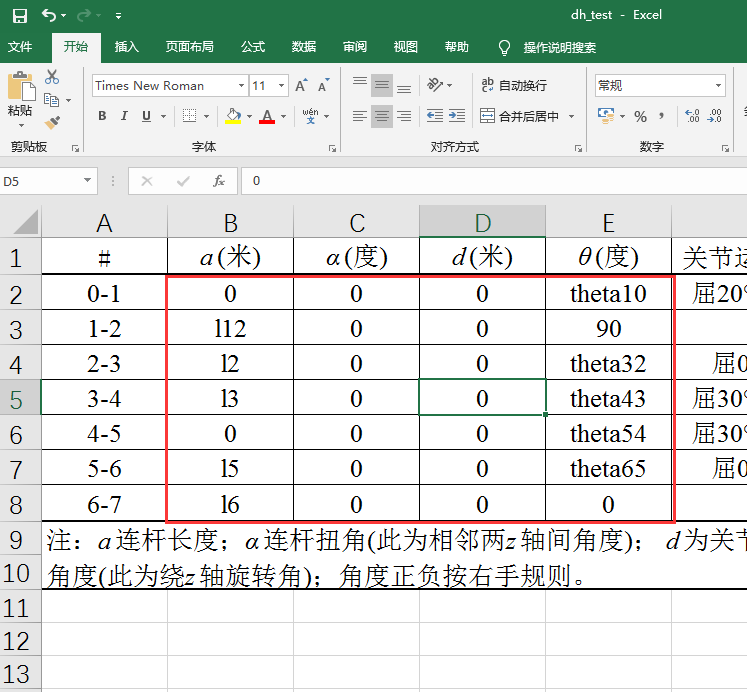那么函数的使用如下：
%%%% 1、首先建立一个DH参数符号矩阵DH_TABLE
syms l12 l2 l3 l5 l6 theta10 theta32 theta43 theta54 theta65 % 定义符号参数
DH_TABLE = [0   , 0, 0, theta10;
l12 , 0, 0, 90;
l2  , 0, 0, theta32;
l3  , 0, 0, theta43;
0   , 0, 0, theta54;
l5  , 0, 0, theta65;
l6  , 0, 0, 0       ] % 定义DH参数表

DH_TABLE =

[   0, 0, 0, theta10]
[ l12, 0, 0,      90]
[  l2, 0, 0, theta32]
[  l3, 0, 0, theta43]
[   0, 0, 0, theta54]
[  l5, 0, 0, theta65]
[  l6, 0, 0,       0]

%%%% 或者直接：DH_TABLE = str2sym('[0,0,0,theta10;l12,0,0,90;l2,0,0,theta32;l3,0,0,theta43;0,0,0,theta54;l5,0,0,theta65;l6,0,0,0]')
%%%% str2sym()函数直接能够生产符号矩阵，但是如果需要带具体数值到所得矩阵公式中计算的话，该定义符号矩阵的方式则不行

%%%% 2、直接调用求DH参数函数求得DH矩阵
>>[Dof,A] = m_SigalMesu(DH_TABLE)

Dof =

7

A(:,:,1) =

[ cos(theta10), -sin(theta10), 0, 0]
[ sin(theta10),  cos(theta10), 0, 0]
[            0,             0, 1, 0]
[            0,             0, 0, 1]

A(:,:,2) =

[ cos(90), -sin(90), 0, l12*cos(90)]
[ sin(90),  cos(90), 0, l12*sin(90)]
[       0,        0, 1,           0]
[       0,        0, 0,           1]

A(:,:,3) =

[ cos(theta32), -sin(theta32), 0, l2*cos(theta32)]
[ sin(theta32),  cos(theta32), 0, l2*sin(theta32)]
[            0,             0, 1,               0]
[            0,             0, 0,               1]

A(:,:,4) =

[ cos(theta43), -sin(theta43), 0, l3*cos(theta43)]
[ sin(theta43),  cos(theta43), 0, l3*sin(theta43)]
[            0,             0, 1,               0]
[            0,             0, 0,               1]

A(:,:,5) =

[ cos(theta54), -sin(theta54), 0, 0]
[ sin(theta54),  cos(theta54), 0, 0]
[            0,             0, 1, 0]
[            0,             0, 0, 1]

A(:,:,6) =

[ cos(theta65), -sin(theta65), 0, l5*cos(theta65)]
[ sin(theta65),  cos(theta65), 0, l5*sin(theta65)]
[            0,             0, 1,               0]
[            0,             0, 0,               1]

A(:,:,7) =

[ 1, 0, 0, l6]
[ 0, 1, 0,  0]
[ 0, 0, 1,  0]
[ 0, 0, 0,  1]


这样便通过DH参数，首先建立一个符号矩阵，再传入求DH矩阵函数，便获得了对应的DH矩阵公式
2）、若需求转换矩阵，同样可直接选择对应的DH矩阵进行相乘等操作获得。如上面方法一中的3)部分代码。
3）、虽然从表面上看来，直接通过excel导入，更加方便。但是对于此求DH矩阵函数而言，由于对excel导入的数据并没进行过多的处理，因而从excel导入的DH参数不可出现特殊字符，也不可能出现  “+”、“-”、“*”、“/”（加、减、乘、除）符号。
！！！！     但是，如果通过建立符号矩阵的方式输入DH参数，参数中可以出现 “+”、“-”、“*”、“/”（加、减、乘、除）运算符号。如下：
%%%% 输入的DH参数具有“*”、“+”运算符号，“l11*l13”、“l12+l2”
>> syms l11 l13 l12 l2 l3 l5 l6 theta10 theta32 theta43 theta54 theta65
>> DH_TABLE = [l11*l13, 0, 0, theta10;
l12+l2 , 0, 0, 90;
l2     , 0, 0, theta32;
l3     , 0, 0, theta43;
0      , 0, 0, theta54;
l5     , 0, 0, theta65;
l6     , 0, 0, 0       ] % 具有加减乘除运算符号的DH参数矩阵

DH_TABLE =
[  l11*l13, 0, 0, theta10]
[ l2 + l12, 0, 0,      90]
[       l2, 0, 0, theta32]
[       l3, 0, 0, theta43]
[        0, 0, 0, theta54]
[       l5, 0, 0, theta65]
[       l6, 0, 0,       0]

>> [Dof,A] = m_SigalMesu(DH_TABLE) % 通过参数矩阵获得DH矩阵

Dof =

7

A(:,:,1) =

[ cos(theta10), -sin(theta10), 0, l11*l13*cos(theta10)]
[ sin(theta10),  cos(theta10), 0, l11*l13*sin(theta10)]
[            0,             0, 1,                    0]
[            0,             0, 0,                    1]

A(:,:,2) =

[ cos(90), -sin(90), 0, cos(90)*(l2 + l12)]
[ sin(90),  cos(90), 0, sin(90)*(l2 + l12)]
[       0,        0, 1,                  0]
[       0,        0, 0,                  1]

A(:,:,3) =

[ cos(theta32), -sin(theta32), 0, l2*cos(theta32)]
[ sin(theta32),  cos(theta32), 0, l2*sin(theta32)]
[            0,             0, 1,               0]
[            0,             0, 0,               1]

A(:,:,4) =

[ cos(theta43), -sin(theta43), 0, l3*cos(theta43)]
[ sin(theta43),  cos(theta43), 0, l3*sin(theta43)]
[            0,             0, 1,               0]
[            0,             0, 0,               1]

A(:,:,5) =

[ cos(theta54), -sin(theta54), 0, 0]
[ sin(theta54),  cos(theta54), 0, 0]
[            0,             0, 1, 0]
[            0,             0, 0, 1]

A(:,:,6) =

[ cos(theta65), -sin(theta65), 0, l5*cos(theta65)]
[ sin(theta65),  cos(theta65), 0, l5*sin(theta65)]
[            0,             0, 1,               0]
[            0,             0, 0,               1]

A(:,:,7) =

[ 1, 0, 0, l6]
[ 0, 1, 0,  0]
[ 0, 0, 1,  0]
[ 0, 0, 0,  1]

>> T = simplify(A(:,:,1)*A(:,:,2)) % 计算0-1-2的转换关系

T =

[ cos(theta10 + 90), -sin(theta10 + 90), 0, cos(theta10 + 90)*(l2 + l12) + l11*l13*cos(theta10)]
[ sin(theta10 + 90),  cos(theta10 + 90), 0, sin(theta10 + 90)*(l2 + l12) + l11*l13*sin(theta10)]
[                 0,                  0, 1,                                                   0]
[                 0,                  0, 0,                                                   1]


这便是通过建立符号矩阵的方式输入DH参数的优点所在！！！
4）、另外，我们在通过matlab获得DH矩阵，甚至进而得到转换矩阵之后；如果需要带入具体数值求值，则需要利用到subs()函数。
subs函数使用格式如下：
subs(表达式，表达式参数，表达式参数对应的新值)
如：求得0-1-2的转换矩阵，并将具体数值带入其矩阵中：
%%%% 获得0-1-2的转换矩阵
>> T = simplify(A(:,:,1)*A(:,:,2))

T =
[ cos(theta10 + 90), -sin(theta10 + 90), 0, cos(theta10 + 90)*(l2 + l12) + l11*l13*cos(theta10)]
[ sin(theta10 + 90),  cos(theta10 + 90), 0, sin(theta10 + 90)*(l2 + l12) + l11*l13*sin(theta10)]
[                 0,                  0, 1,                                                   0]
[                 0,                  0, 0,                                                   1]

%%%% 将l12 = 2 带入T矩阵
>> t = subs(T,l2,2)

t =
[ cos(theta10 + 90), -sin(theta10 + 90), 0, cos(theta10 + 90)*(l12 + 2) + l11*l13*cos(theta10)]
[ sin(theta10 + 90),  cos(theta10 + 90), 0, sin(theta10 + 90)*(l12 + 2) + l11*l13*sin(theta10)]
[                 0,                  0, 1,                                                  0]
[                 0,                  0, 0,                                                  1]

%%%% 将theta10 = 90°，l11 = 1，l12 = 2 ,l2 = 3，l3 = 0.5 带入T矩阵
>> t = subs(T,[theta10 l11 l12 l2 l3],[90 1 2 3 0.5])

t =
[ cos(180), -sin(180), 0, 5*cos(180) + l13*cos(90)]
[ sin(180),  cos(180), 0, 5*sin(180) + l13*sin(90)]
[        0,         0, 1,                        0]
[        0,         0, 0,                        1]


注：本次利用到了matlab符号函数计算工具，具体的相关知识参考：
https://wenku.baidu.com/view/e488e5decc7931b764ce15c4.html


展开全文matlab应用 matlab符号函数
• ## DH参数

千次阅读 2017-12-11 18:34:50
• DH参数 a d θ α a：连杆长度 d：连杆偏置 θ：关节角度 α：连杆扭曲 存在性和唯一性问题 如果两个坐标系之间存在如下特性： （DH1）坐标轴X1垂直于坐标轴Z0。 （DH2）坐标轴X1与坐标轴Z0相交。 则存在唯一的a d ...
DH参数
a d θ α
a：连杆长度
d：连杆偏置
θ：关节角度
α：连杆扭曲
存在性和唯一性问题
如果两个坐标系之间存在如下特性：
（DH1）坐标轴X1垂直于坐标轴Z0。
（DH2）坐标轴X1与坐标轴Z0相交。
则存在唯一的a d θ α有可将参考系1中的坐标变换到参考系0中。
坐标系示意图说明
参数a：轴Z0和轴Z1之间沿着轴线X1测得的距离。
角度α：在垂直于X1的平面内测得的轴线Z0和Z1之间的夹角。角度α的正向取值定义为从Z0到Z1，旋转方向符合右手规则。
参数d：从原点O0到轴线X1与Z0交点之间的距离，该距离沿Z0轴线进行测量得到。
参数θ：在垂直于Z0的平面内测得的从X0到X1的角度。
（坐标系的配置应该设法满足DH1和DH2约束条件）

连杆
a i
Alpha i
d i
Theta i

1

2

3

某一连杆的DH参数矩阵参考：
机器人建模和控制：马克 W.斯庞.机械工业出版社


展开全文线性代数
• 六自由度机械臂研究（3）- 机械臂DH参数表建立 DH参数简介 DH参数全称是Denavit-Hartenberg参数， 目前是机器人领域广泛应用于机器人结构描述的方法。 我们可以用这些参数来来描述一个机器人机构， 并用这些参数来...六自由度机械臂 教程
• ## DH参数介绍

万次阅读 2018-05-25 09:39:07
机械手末端到基坐标系的变换关系： 通常，每一个变换需要６个独立参数来描述坐标系ｉ相对坐标系ｉ－１的关系，３个用来描述位置另外３个用来描述...ＤＨ参数第一次提出来以后经过了几次修改。以下是几个代表性的...
• ## DH参数的理解

千次阅读 2020-03-05 15:01:48
根据轴的先后顺序分为标准DH参数和改进DH参数。 标准DH参数：两个连杆坐标系变换是先绕z轴转动、平移，再绕x轴转动、平移。即X-Z顺序，先转动后平移。 改进DH参数：两个连杆坐标系变换是先绕X轴转动、平移，再绕Z轴...
• ## ＤＨ参数分配算法

千次阅读 2018-05-25 10:17:39
具有n个自由度（即关节）的开放式运动链的参数分配过程总结如下： 从{1，2，…，n}标记所有关节。 从{0，1，…，n}标记所有链接，0表示为固定链接。 绘制线条通过所有关节，定义关节轴。 将每个坐标系的Z轴指定为...
• 影响 对于树形结构或者闭链机构的机器人来说，按照SDH方法建立的连杆坐标系会产生歧义，因为SDH的建系原则是把连杆i的坐标系建立在连杆的远端，如图3(a)所示，这就导致连杆0上同时出现了两个坐标系。...
• ## DH参数与运动学

千次阅读 2017-10-09 22:46:19
我们使用矩阵相乘表示机械臂末端中心点(Tool Central Point, TCP)，如 M60=T10T21T32T43T54T65 M_0^6= T_0^1 T_1^2 T_2...还可以利用变换矩阵的特殊性质进行化简DH参数的完整表达UR系列机械别的DH参数见附录数学公式使
• 机械手臂的一个重要特征参数就是DH参数。具体资料可查询：维基百科英文版：http://en.wikipedia.org/wiki/Denavit%E2%80%93Hartenberg_parameters由于被墙，现摘录如下： Denavit–Hartenberg parameters From ...
• 对于一个机械臂，这里已旋转副连接为例，描述需要用到两个参数，连杆转角和连杆长度。这连个都是机械臂本身结构决定的参数。用于描述机械臂两端连接轴的数学关系，转角和公垂线的距离 在用于描述两个机械臂之间的...人工智能
• DH参数 DH参数（Denavit–Hartenberg parameters）是一个用四个参数表达两对关节连杆之间位置角度关系的机械臂数学模型和坐标系确定系统。 DH选的四个参数都的含义如下： link length（连杆长度）：两个关节的轴...
• 建议先阅读&amp;amp;lt;上一篇&amp;amp;gt;。 ...此处说到的SCARA机器人是KUKA KR10机器人： ...它是一个revolute_revolute_prismatic_revolute结构或者简称为RRPR结构，并且所有的关节轴都是平行的。...
• 最近做了一下PUMA560的轨迹规划，发现它的DH参数多种多样...链接:简直要崩溃了，PUMA560机器人DH参数表，你们见过多少个版本？. 先按这个同学列出的4个不同形式的参数来分析，我把这4个归类为3种。 原因有下： 1、如...
• 根据DH建模标定后的参数代入下式，可以获得坐标系i中的点在i-1坐标系中的描述。 对于整个机械臂这可以通过每个关节的转换矩阵进行转换 从关节角度可以推倒出工作空间坐标，也可以推导驱动器的执行命令。这样...
• 如何获取ABB机器人DH参数 以查找ABB IRB1200的DH参数为例 如果电脑中安装有RobotStudio，则可以直接进行获取ABB机器人DH参数； 进入安装目录下%\ABB Library\Robots\IRB1200FPL_5_90_STD_01.rslib； 用解压软件打开......